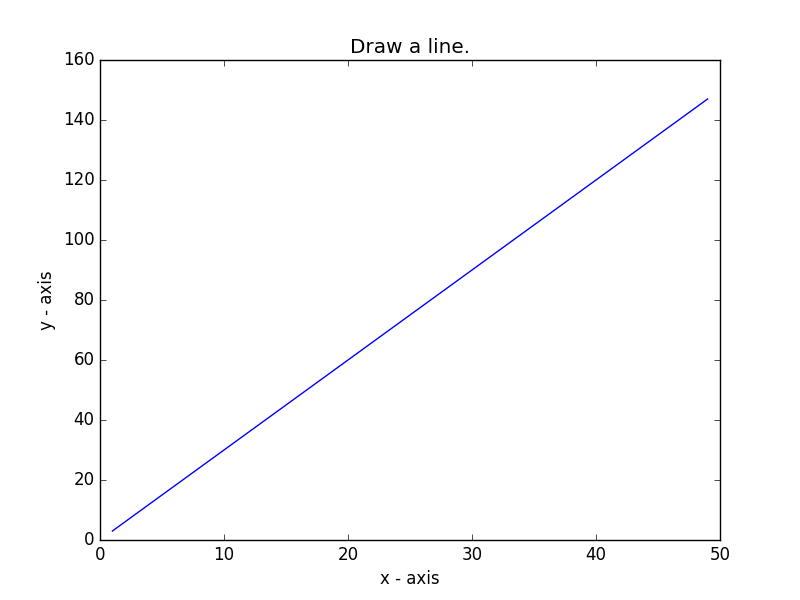﻿ Matplotlib Basic: Draw a line with suitable label in the x axis, y axis and a title - w3resource# Matplotlib Basic: Draw a line with suitable label in the x axis, y axis and a title

## Matplotlib Basic: Exercise-1 with Solution

Write a Python program to draw a line with suitable label in the x axis, y axis and a title.

Sample Solution:

Python Code:

``````import matplotlib.pyplot as plt
X = range(1, 50)
Y = [value * 3 for value in X]
print("Values of X:")
print(*range(1,50))
print("Values of Y (thrice of X):")
print(Y)
# Plot lines and/or markers to the Axes.
plt.plot(X, Y)
# Set the x axis label of the current axis.
plt.xlabel('x - axis')
# Set the y axis label of the current axis.
plt.ylabel('y - axis')
# Set a title
plt.title('Draw a line.')
# Display the figure.
plt.show()
```
```

Sample Output:Python Code Editor: